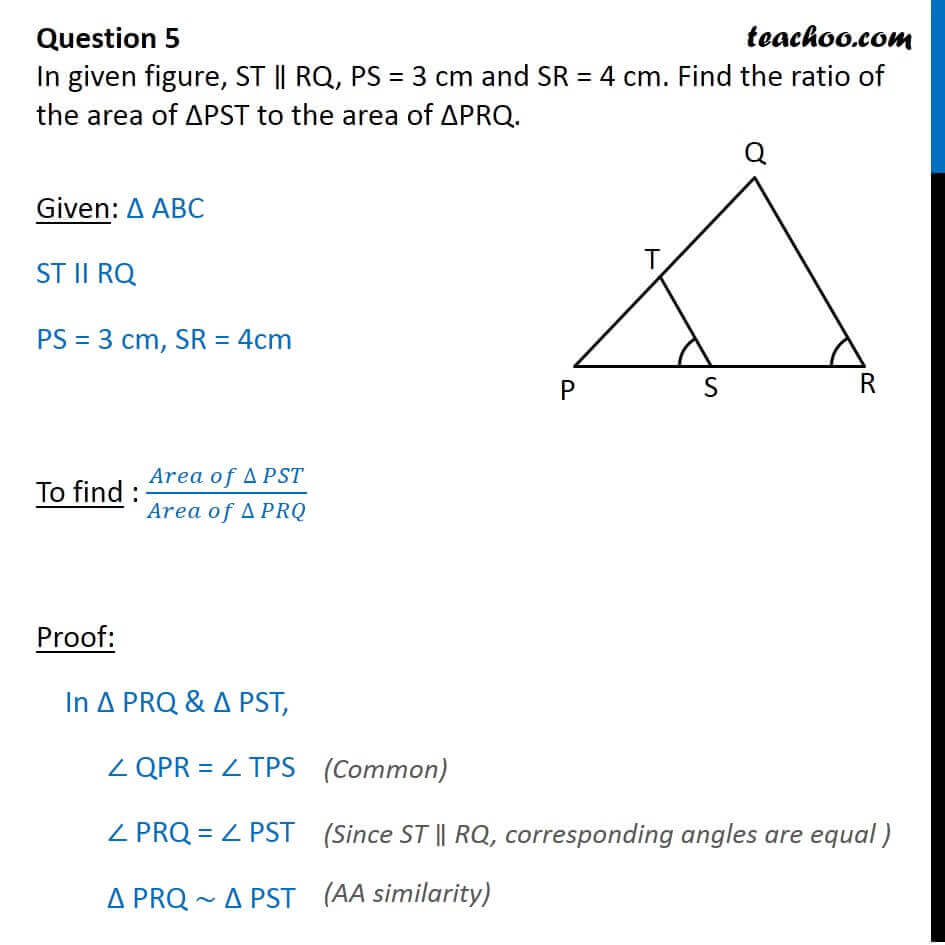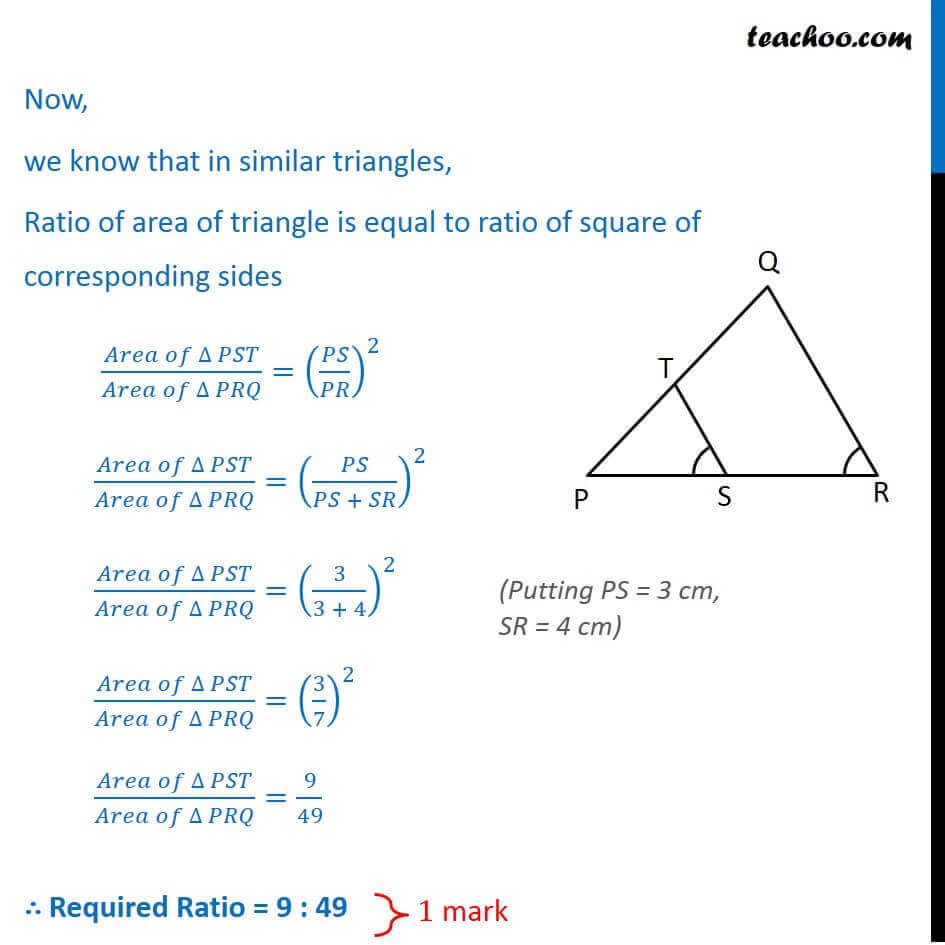CBSE Class 10 Sample Paper for 2018 Boards

Class 10
Solutions of Sample Papers for Class 10 Boards

### In given figure, ST ∥ RQ, PS = 3 cm and SR = 4 cm. Find the ratio of the area of ∆PST to the area of ∆PRQ.

This is a question of CBSE Sample Paper - Class 10 - 2017/18.Get live Maths 1-on-1 Classs - Class 6 to 12

### Transcript

Question 5 In given figure, ST RQ, PS = 3 cm and SR = 4 cm. Find the ratio of the area of PST to the area of PRQ. Given: ABC ST II RQ PS = 3 cm, SR = 4cm To find : ( )/( ) Proof: In PRQ & PST, QPR = TPS PRQ = PST PRQ ~ PST Now, we know that in similar triangles, Ratio of area of triangle is equal to ratio of square of corresponding sides ( )/( )=( / )^2 ( )/( )=( /( + ))^2 ( )/( )=(3/(3 + 4))^2 ( )/( )=(3/7)^2 ( )/( )=9/49 Required Ratio = 9 : 49 (Putting PS = 3 cm, SR = 4 cm)